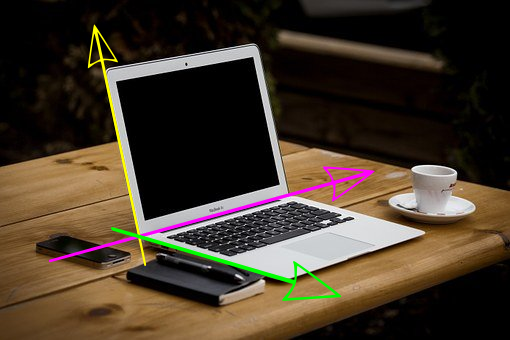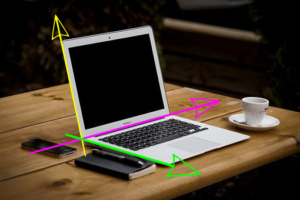# What does it mean to say orthogonal?

In this article, let us explore what is meant by the concept of orthogonality.

Orthogonal or orthogonality is often introduced from a mathematical perspective. In general, most resources explains it in terms of linear algebra and vectors.

One such example is when we learn about right-angled triangles the two sides are drawn as perpendicular or orthogonal to each other.

The perpendicular perspective serves the purpose in simple examples but I often see more story behind the orthogonality concept. Let us start by thinking about the space in which where we represent such orthogonal vectors.

In the figure above the two axes, $x$ and $y$ are drawn in a 2D plane. We also can extend the same concept to a three-dimensional real world where we can represent three vectors perpendicular to each other with an angle of $90^{\circ}$.

Please not that here I am trying to represent 3D space in 2D. Also the laptop screen is assumed perpendicular to the table.Here I have marked the laptop’s screen direction as one dimension, keyboard direction as another one, and possibly the position of the ports as the third.

If we go and measure the angle between any of the green, yellow, or purple axes will give us $90^{\circ}$.

Here no part of one axis is composed of any other axes. This means that all of those vectors were independent of each other.

What about vectors of more than three components?. Can we say they are also orthogonal just because the angle between them is $90^{\circ}$?.

Forget measuring angle. How do we even represent a space higher than three dimension?

We can immediately see how we are limited by the three-dimensional world and struggling hard to connect the n-dimensional idea to some physical reality.

In science and engineering, we will be dealing with n-dimensional vectors. This is why we need a better intuitive understanding of orthogonality rather than a mathematical rigorous one.

### The intuition for orthogonality

Let me explain it through a story of a loving couple. There were a husband and wife who loved each other very much.However, they were financially struggling.

Once their wedding anniversary was approaching and both the couples decided to give a surprising gift to the spouse.

The wife remembered her husband had a vintage watch and its strap needed a replacement. The husband was putting off the repairment due to their bad financial conditions.

She decided to gift him a new strap. In the meantime, the husband was thinking of how his wife has adorable hair and knew she wanted to buy an expensive comb.

What happened was the husband sells the watch and buys the expensive comb as a gift to his wife. The wife donates hair for money and buys him a new watch strap.

They did these independently without telling the other to make it a surprise.

We can think of the interaction of their actions were canceling the purpose of each other and the net effect was none!.

If we connect this idea to mathematical terms, we can see that even though the individual components were non-zero, the interaction of n-components of two vectors can essentially cancel each other. This happens when the total action of the vectors is nullifying each other.

Rather than trying to see the concept as a simple geometric relation which only works in a 2D or 3D world, we now have a big picture for orthogonality.

Higher-order mathematics can assume $k$ orthogonal components where every component is orthogonal to every other.

The concept of orthogonal functions or signals had tremendous application in signal decomposition, dimensionality reduction, etc.

The prime application is decomposing a complex thing to its fundamental components. Infact, it is the independence and orthogonality properties are making the components fundamental. This will help us to understand, process, and make use of the complex enity easily.

### Few Applications

If you are from an electrical engineering and computer science background, you might know communication systems heavily employ the orthogonality for signal encoding. Orthogonal Frequency Division Multiplication (OFDM) is one such application.

One other use case is sequence alignment where researchers used four complex numbers ($1+0i$, $-1+0i$, $0+1i$, and $0-1i$ ) to represent nucleotide bases and do the alignment.

### Conclusion

Orthogonality is yet another fundamental building block to our thinking toolset. We will see in other posts how this orthogonality idea recurs in other concepts. We will upgrade our intuitions as we work on new concepts that use it.